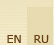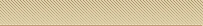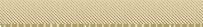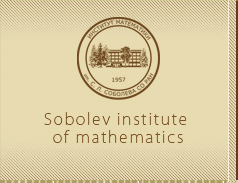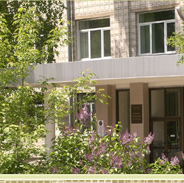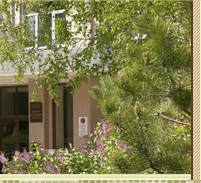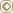MainWeb-postSite mapS. L. S o b o l e vPublicationsS e r g e i  L'v o v i c h  S o b o l e v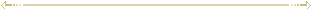Publications  (1962-1963) Some applications of functional analysis in mathematical pfysics (Russian) — Novosibirsk, 1962. — 255 pp. — (Acad. Sci. USSR Siberian Branch). Einige Anwendungen der Funktionalanalysis auf Gleichungen der mathematischen Physik (German) — Berlin: Akademie-Verlag, 1964. — 218 s. MR0172144 (30 #2370) To readers (Russian) // Numerical methods of optimal planning. — Novosibirsk, 1962. — P. 3-5. — With  G. A. Prudenskii. The mathematical problems of modern cybernetics (Russian) // Akad. Nauk SSSR Izv. Sibirsk. Otd. — 1962. — No. 5. — P. 3-13. — With A. A. Ljapunov. MR0151338 (27 #1324) Cubature formulas on the sphere which are invariant under transformations of finite rotation groups (Russian) // Dokl. Akad. Nauk SSSR. — 1962. — Vol. 146, No. 2. — P. 310-313. MR0141225 (25 #4635) Cubature formulas on the sphere which are invariant under finite groups of rotations (English) // Soviet Math. Dokl. — 1962. — Vol. 3. — P. 1307-1310. Zbl. 119.28701 Über Formeln der mechanischen Kubatur auf e einer Kugelfläche (Russian) // Sib. Mat. Zh. — 1962. — Vol. 3, No. 5. — P. 769-796. Zbl. 202.44501 The formulas of mechanical cubature on the surface of a sphere (Russian) // Sibirsk. Mat. Zh. — 1962. — Vol. 3, No. 5. — P. 769-796. MR0141227 (25 #4637) On the number of nodes in cubature formulae on a sphere (Russian) // Dokl. Akad. Nauk SSSR. — 1962. — Vol. 146, No. 4. — P. 770-773. MR0141226 (25 #4636) The number of nodes in cubature formulas on the sphere (English) // Sov. Math. Dokl. — 1962. — Vol. 3. — P. 1391-1394. Zbl. 119.28702 Various types of convergence and quadrature formulas (Russsian) // Dokl. Akad. Nauk SSSR. — 1962. — Vol. 146, No. 1. — P. 41-42. MR0140184 (25 #3606) Axioms and paradoxes of education: [On the problems of scientific staff training] (Russian) // Unost’. — 1962. — No. 6. — P. 65-70. Yes, it is quite serious! [On the discussion about cybernetics] (Russian) // Lit. Gazeta. — 1962. — June 2. Each scientist is a propagandist of science (Russian) // Sov. Rossiya. — 1962. — July 5. Mathematics today and tomorrow (Russian) // Molodost’ Sibiri. — 1962. — January 28. Optimal strategy: [On the development of computer technology] (Russian) // Ogonek. — 1962. — No. 48. — P. 17-18. A letter to the editor [concerning Yu.V.Knorozov’s paper «Computer decipherment of the Maya writing language»] (Russian) // Vopr. yazikoznaniya. — 1962. — No. 1 // Vopr. yazikoznaniya. — 1962. — No. 3. — P. 147. To teach to think: [On scientific staff training and tasks of scientific schools] (Russian) // Lit. Gazeta. — 1962. — June 26. Some questions of the theory of cubature formulas (Russian) — Novosibirsk, 1963. — 8 pp. — (Acad.Sci. USSR Siberian Branch. Outlines Joint Soviet-American Sympos. on Partial Differential Equations, 1963, Novosibirsk). Some questions of the theory of cubature formulas (English) // Joint Soviet-American Sympos. on Partial Differential Equations, 1963, Novosibirsk: Outlines. — Moscow, 1963. — P. 241-245. Über Kubaturformeln (Russian) // Stud. Math., Ser. Spec. — 1963. — No. 1. — P. 117-118. Zbl. 115.27801 On cubature formulae (Russian) // Studia Math. (Ser. Specjalna.) Zeszyt — 1963. — No. 1. — P. 117-118. MR0145659 (26 #3188) A method of calculating coefficients for mechanical cubature formulae (Russian) // Dokl. Akad. Nauk SSSR. — 1963. — Vol. 150, No. 6. — P. 1238-1241. MR0150951 (27 #937) A method for calculating the coefficients of mechanical cubature formulas (English) // Soviet Math. Dokl. — 1963. — Vol. 4. — P. 878-882. Zbl. 237.65018 The density of compactly supported functions in the space Lpm(En) (Russian) // Dokl. Akad. Nauk SSSR. — 1963. — Vol. 149, No. 1. — P. 40-43. MR0149268 (26 #6758) The density of finite functions in the Lpm(En) space (English) // Soviet Math. Dokl. — 1963. — Vol. 4. — P. 313-316. Zbl. 194.14901 Die Dichte der finiten Fuktionen im Raum Lpm(En) (Russian) // Sib. Mat. Zh. — 1963. — Vol. 4, No. 3. — P. 673-682. Zbl. 204.43802 The density of compactly supported functions in the space Lpm(En) (Russian) // Sibirsk. Mat. Zh. — 1963. — Vol. 4, No. 3. — P. 673-682. MR0174966 (30 #5156) Einbettungssätze (Russian) // Tr. Chetvertogo Vsesoyuzn. Mat. S«ezda (Leningrad, 1961) — Vol. 1. — Leningard, 1963. — P. 227-242. — With S. M. Nikol’skij. Zbl. 204.13602 Embedding theorems (Russian) // Proc. Foyrth All-Union Math. Congr. (Leningrad, 1961) — Vol. 1. — Leningrad, 1963. — P. 227-242. — With S. M. Nikol’skii. MR0171188 (30 #1419) Imbedding theorems (English) // Amer. Math. Soc. Transl. Ser. 2. — 1970. — Vol. 87. — P. 147-173. Zbl. 206.12204 Equations aux derivees partielles pour les fonctions extremales des problemes du calcul numerique a plusieurs variables independantes (French) // Les Equations aux Derivees Partielles. — Paris, 1963. — P. 197-206. Zbl. 234.65029 MR0165703 (29 #2983) Some new problems in the theory of partial differential equations (English) // Differential Equations and Their Applications: Proc. Conf., — Prague, 1962. — Prague, 1963. — P. 167-177. Zbl. 161.08603 MR0169392 (29 #6642) Sur les problemes mixtes pour les equations aux derivees partielles a deux variables independantes // Calcutta Math. Soc. Golden Jubilee Commemoration. — Calcutta, 1963. — Vol. (1958/59), Part. 2. — P. 447-484. Great contribution into mathematics (Russian) // Sov. Rossiya. — 1963. — March 8. The torch of talent. Development of mathematics and scientific staff training (Russian) // Izvestiya. — 1963. — March 24. — With  M. A. Lavrent’ev et al.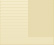©Sobolev Institute of Mathematics, 2009      Siberian Branch of the Russian Academy of Sciences4 Acad. Koptyug avenue, 630090 Novosibirsk Russia Tel.: (383) 333-28-92 Fax: (383) 333-25-98 E-mail: